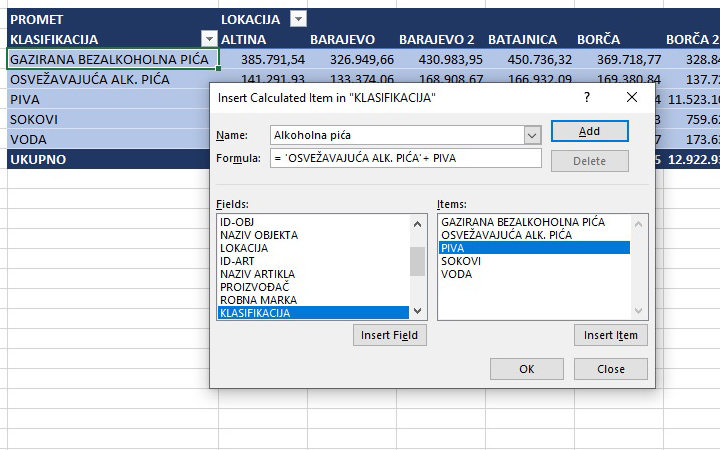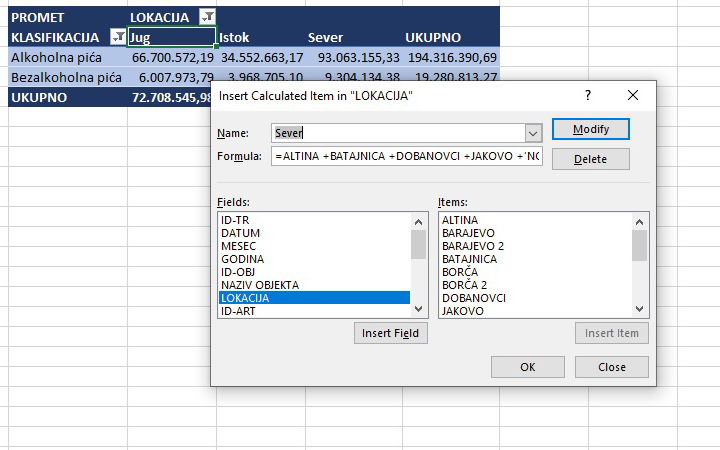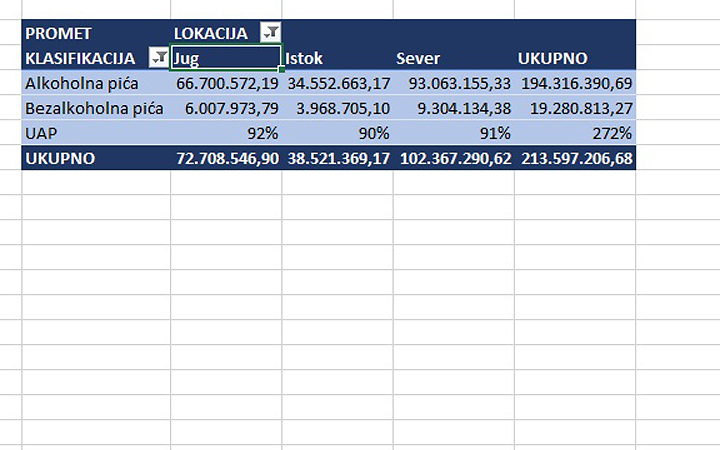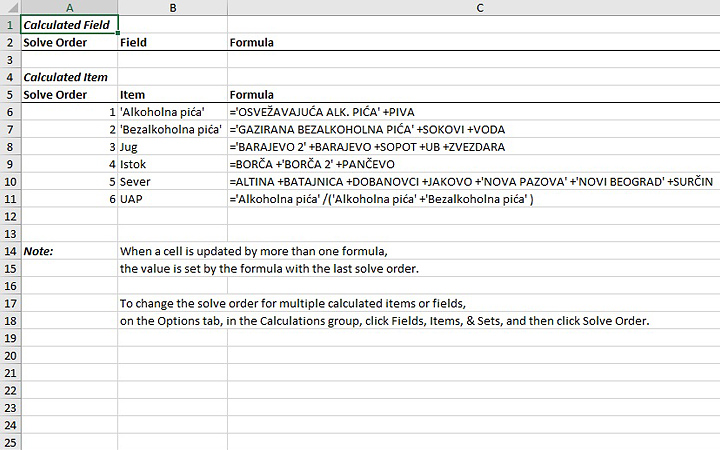# Solve Order

Calculated Items allow you to create formulas based on arithmetic operations between elements in rows and/or columns within a pivot table. Sometimes Excel can get confused and display incorrect results due to a misinterpretation of the order of computational operations. Solve order is an option with which we can change the order to get the correct result.

We will start with, previously made, a pivot table showing sales in relation to the classification (rows) and locations of retail facilities (columns). How to create a Calculated Item? If e.g. click on the first element of the Rows field of a pivot table (carbonated soft drinks), go to the Analyze ribbon, and in the Fields, Items & Sets menu, select Calculated Item, a window will open for creating the formula of the calculated element. In the Name field, enter the name, and in the Formula field, the expression with which the calculation is performed, and represents the arithmetic operations with between the elements shown in the list on the right side of the window. Eg. Alcoholic beverages (Alkoholna pića) would be the sum of the elements Refreshing Alcoholic Beverages and Beers. The sum of the remaining elements can be used to create a calculated element for soft drinks (Bezalkoholna pića).In a similar way, we can add the calculated elements South (Jug), North (Sever) and East (Istok) for the locations of retail facilities depending on their geographical determinants.It is also possible to create formulas for calculated elements based on previously created formulas. The share of alcoholic beverages in the total amount is calculated according to the formula:

UAP= ‘Alkoholna pića’/( ‘Alkoholna pića’+ ‘Bezalkoholna pića’)

If we have created the calculated elements in the previous order, a calculation error may occur. This is because, instead of calculating percentages, Excel adds values ​​(shares) by location. To get an accurate calculation, we need to change the order of calculation. This is done by going to the Analyze ribbon, so in the Fields, Items & Sets menu, select Solve Order. Here we will see all the formulas for obtaining the calculated elements. The UAP formula should be moved to the bottom of the table. After we perform this action, the formula for the share of alcoholic beverages in the total turnover will show the correct result.There is also a List Formulas option in the previous menu. If we run it Excel will create a new worksheet in which it will list all the formulas we have created to get the calculated elements.